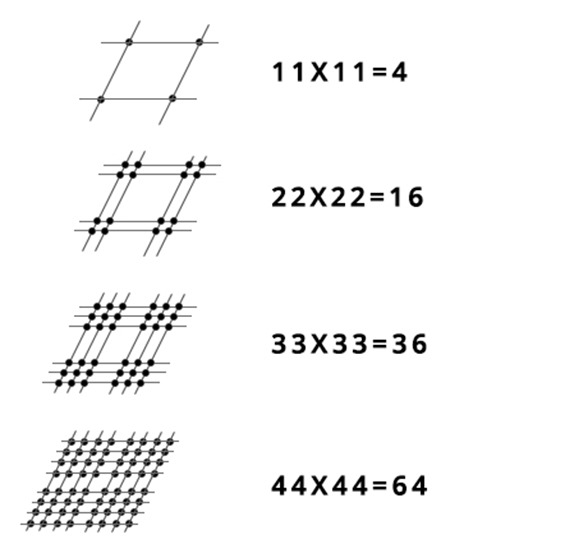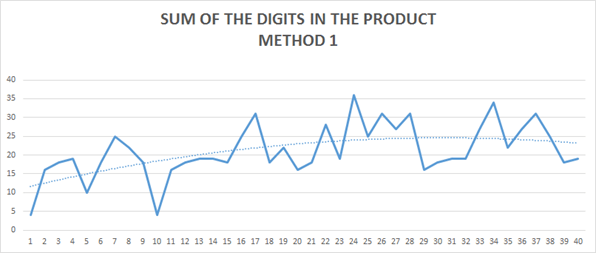# Only a Math Genius can Solve this Puzzle–Not Really!One of the most popular math equation puzzles on social media is interesting because it doesn’t have one correct answer and it illustrates the nature of a solution divergence.

Here is an example. The following two problems can be solved correctly regardless if we use sum of the digits in the product or product of the sum of digits methods:

Set 1: 11×11=4
Set 2: 22×22=16
Set 3: 33×33=?

For first set
Method 1   11×11=121 then summing the digits in the products give us 1+2+1=4
Method 2   (1+1) x (1+1) = 4

and for second set
Method 1  22×22=484 then summing the digits in the product gives us 4+8+4=16
Method 2  (2+2) x (2+2) = 16

But when it comes to the next set of 33×33=? each solution diverges and will yield two different results (see result bellow for method 1 and 2).

Method 1 (sum of the digits in the product) it is: 33×33=18

33×33=1089 or 1+0+8+9= 18

Method 2 (product of the sum of digits) it is: 33×33=36

(3+3) x (3+3) = (6) x (6)=36

Here is a graphic solution for method 2Here are the solution for the first 40 sets for each method.

 Method 1 Method 2 11 11 121 4 11 11 4 22 22 484 16 22 22 16 33 33 1089 18 33 33 36 44 44 1936 19 44 44 64 55 55 3025 10 55 55 100 66 66 4356 18 66 66 144 77 77 5929 25 77 77 196 88 88 7744 22 88 88 256 99 99 9801 18 99 99 324 110 110 12100 4 110 110 400 121 121 14641 16 121 121 484 132 132 17424 18 132 132 576 143 143 20449 19 143 143 676 154 154 23716 19 154 154 784 165 165 27225 18 165 165 900 176 176 30976 25 176 176 1024 187 187 34969 31 187 187 1156 198 198 39204 18 198 198 1296 209 209 43681 22 209 209 1444 220 220 48400 16 220 220 1600 231 231 53361 18 231 231 1764 242 242 58564 28 242 242 1936 253 253 64009 19 253 253 2116 264 264 69696 36 264 264 2304 275 275 75625 25 275 275 2500 286 286 81796 31 286 286 2704 297 297 88209 27 297 297 2916 308 308 94864 31 308 308 3136 319 319 101761 16 319 319 3364 330 330 108900 18 330 330 3600 341 341 116281 19 341 341 3844 352 352 123904 19 352 352 4096 363 363 131769 27 363 363 4356 374 374 139876 34 374 374 4624 385 385 148225 22 385 385 4900 396 396 156816 27 396 396 5184 407 407 165649 31 407 407 5476 418 418 174724 25 418 418 5776 429 429 184041 18 429 429 6084 440 440 193600 19 440 440 6400It is interesting to note the series growth patterns for each method.  Where in method 1, the values tend to cluster around a range of several values (see pattern for 30K solutions), in method 2 the growth is polynomial.